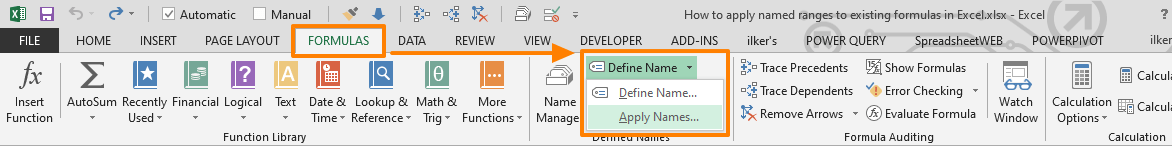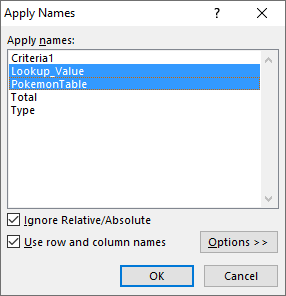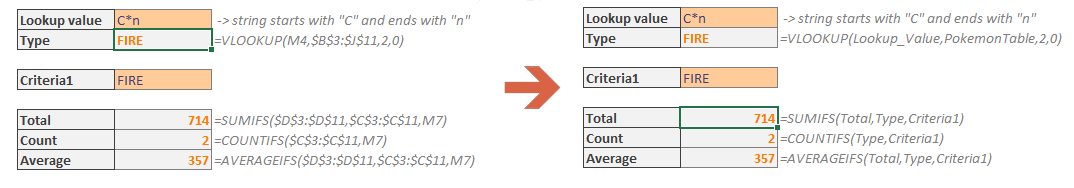The use of named ranges is a very powerful tool for creating references in Excel. In addition to making formulas easier to read, you don't have to remember the exact cell and range references when writing formulas. If you have already been using named ranges, congratulations! You have a very neat workbook. If you want to get started and use this feature in an existing workbook, you do not need to update each reference one-by-one. In this guide, we're going to show you how to apply named ranges to existing formulas in Excel.

Let's see the steps to apply named ranges to existing formulas.

1. Start by defining your named ranges. If you need some pointers, see 5 Ways to Create an Excel Named Range.2. Select the cell or cells that contain formulas. If you do not want to update references in a formula, you can skip those cells.

In our case, we selected the cell M5, which contains the following formula:

=VLOOKUP(M4,\$B\$3:\$J\$11,2,0)1. Activate the Formulas tab in the Ribbon.
2. Click on the arrow next to Define Name and click Apply Names.1. Apply Names dialog will appear. Select the named ranges you want to replace with references.

We selected Lookup_Value (\$M\$4) and PokemonTable (\$B\$3:\$J\$11) names.1. Click OK to apply the named ranges to existing formulas.The formula becomes:

=VLOOKUP(Lookup_Value,PokemonTable,2,0)